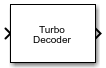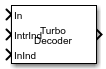# Turbo Decoder

Decode input signal using parallel concatenated decoding scheme

•Libraries:
Communications Toolbox / Error Detection and Correction / Convolutional

## Description

The Turbo Decoder block decodes the input signal using a parallel concatenated decoding scheme. The iterative decoding scheme uses the a posteriori probability (APP) decoder as the constituent decoder, an interleaver, and a deinterleaver. The two constituent decoders use the same trellis structure and decoding algorithm. For more information, see Parallel Concatenated Convolutional Decoding Scheme and APP Decoder.

This icon shows the block with all ports enabled.## Ports

### Input

expand all

Parallel concatenated codeword, specified as a column vector of length M, where M is the length of the parallel concatenated codeword.

Data Types: `double` | `single`

Interleaver indices, specified as a column vector of integers. The vector must be of length L. Each element of the vector must be an integer in the range [1, L] and must be unique. L is the length of the decoded binary output message, `Out`. The interleaver indices define the mapping used to permute the input bits at the decoder.

#### Dependencies

To enable this port, set the Source of interleaver indices parameter to ```Input port```.

Data Types: `double`

Input indices for the bit ordering and puncturing used on the fully encoded data, specified as a column vector of integers. The length of the InInd vector must equal the length of the input data vector In. Element values in the vector must be relative to the fully encoded data for the coding scheme, including the tail bits for all streams.

#### Dependencies

To enable this port, set the Source of input indices parameter to `Input port`.

Data Types: `double`

### Output

expand all

Decoded message, returned as a binary column vector of length L, where L is the length of the decoded binary output message. This output inherits its data type from the `In` input.

## Parameters

expand all

Specify the trellis as a MATLAB® structure that contains the trellis description for a rate KN constituent convolutional code. K is the number of input bit streams, and N is the number of output bit streams.

Note

K must be 1 for the turbo coder. For more information, see Coding Rate.

You can either use the `poly2trellis` function to create the trellis structure or create it manually. For more about this structure, see Trellis Description of a Convolutional Code and the `istrellis` function.

The trellis structure contains these fields.

Number of symbols input to the encoder, specified as an integer equal to 2K, where K is the number of input bit streams.

Number of symbols output from the encoder, specified as an integer equal to 2N, where N is the number of output bit streams.

Number of states in the encoder, specified as a power of 2.

Next states for all combinations of current states and current inputs, specified as a matrix of integers. The matrix size must be `numStates` by 2K.

Outputs for all combinations of current states and current inputs, specified as a matrix of octal numbers. The matrix size must be `numStates` by 2K.

Specify the source of the interleaver indices as `Property` or ```Input port```.

• When you set this parameter to `Property`, the block uses the Interleaver indices parameter to specify the interleaver indices.

• When you set this parameter to ```Input port```, the block uses the `IntrInd` input port to specify the interleaver indices.

Specify the interleaver indices that define the mapping used to permute codeword bits input to the decoder as a column vector of integers. The vector must be of length L. Each element of the vector must be an integer in the range [1, L] and must be unique. L is the length of the decoded binary output message.

#### Dependencies

To enable this parameter, set the Source of interleaver indices parameter to `Property`.

Specify the source of the input indices as `Auto`, `Property`, or `Input port`.

• When you set this parameter to `Auto`, the block computes input indices that assume the second systematic stream is punctured and all tail bits are included in the input.

• When you set this parameter to `Property`, the block uses the input indices that you specify for the Input indices parameter.

• When you set this parameter to ```Input port```, the block uses the InInd input port to specify the input indices. The vector length and values for the input indices and coded input signal can change with each execution of the block.

Specify the input indices for the bit ordering and puncturing used on the fully encoded data as a column vector of integers. The vector length of this parameter must equal the length of the input data vector In.

#### Dependencies

To enable this parameter, set the Source of input indices parameter to `Property`.

Specify the decoding algorithm that the constituent APP decoders use to decode the input signal as `True APP`, `Max*`, `Max`. When you set this parameter to `True APP` the block implements true a posteriori probability decoding. When you set this parameter to `Max*` or `Max` the block uses approximations to increase the speed of the computations. For more information, see APP Decoder.

Specify the number of bits which the constituent APP decoders must use to scale the input data to avoid losing precision during computations as an integer in the range [0, 8]. The constituent decoders multiply the input by 2k and divide the pre-output by the same factor. k is the value of the Number of scaling bits parameter. For more information, see APP Decoder.

#### Dependencies

This enable this parameter, set the Decoding algorithm parameter to `Max*`.

Specify the number of decoding iterations the block uses as a positive integer. The block iterates and provides updates to the log-likelihood ratios (LLR) of the uncoded output bits. The output of the block is the hard-decision output of the final LLR update.

Type of simulation to run, specified as ```Interpreted execution``` or `Code generation`.

• `Interpreted execution` — Simulate the model by using the MATLAB interpreter. This option requires less startup time, but the speed of subsequent simulations is slower than with the ```Code generation``` option. In this mode, you can debug the source code of the block.

• `Code generation` — Simulate the model by using generated C code. The first time you run a simulation, Simulink® generates C code for the block. The model reuses the C code for subsequent simulations unless the model changes. This option requires additional startup time, but the speed of the subsequent simulations is faster than with the `Interpreted execution` option.

## Block Characteristics

 Data Types `double` | `single` Multidimensional Signals `no` Variable-Size Signals `yes`